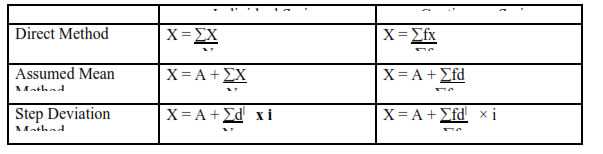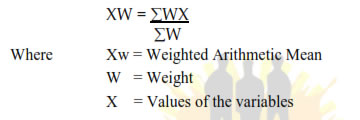Chapter 5 Measures of Central Tendency

## Important Term and Concepts:

1. Average: It is a value which is typical or representative of a set of data. Averages are also called Measures of Central Tendency.

2. Functions of Average:

i] Presents complex data in a simple form.
ii] Facilitates comparison.
iii] Helps government to form policies.
iv] Useful in Economic analysis.

3. Essentials of a good Average:

i. Simple to calculate.
ii. It should be easy to understand.
iii. Rigidly defined.
iv. Based on all items of observation.
v. Least affected by extreme values.
vi. Capable of further algebraic treatment.
vii. Least affected by sampling fluctuation.
viii. Graphic measurement possible.

4. Types of Averages:

i. Arithmetic Mean
ii. Median
iii. Mode
iv. Quartiles

5. Arithmetic Mean (X)

It is the most common type of measures of central tendency. It is obtained by dividing the sum of all observation in a series by the total number of observation.

6. Calculation of Arithmetic Mean:7. Merits of Arithmetic Mean:

1] Easy to calculate
2] Simple to understand
3] Based on all observations
4] Capable of further mathematical calculations.

Demerits :

1] Affected by extreme values.
2] Cannot be calculated in open-end series.
3] Cannot be graphically ascertained.
4] Sometimes misleading or absurd result.

8. Weighted Arithmetic Mean:

Values to be arranged are given varying importance.9. Median (M)

It is defined as the middle value of the series, when the data is arranged in ascending or descending order.

### NCERT Books Free Pdf Download for Class 5, 6, 7, 8, 9, 10 , 11, 12 Hindi and English Medium

 Mathematics Biology Psychology Chemistry English Economics Sociology Hindi Business Studies Geography Science Political Science Statistics Physics Accountancy